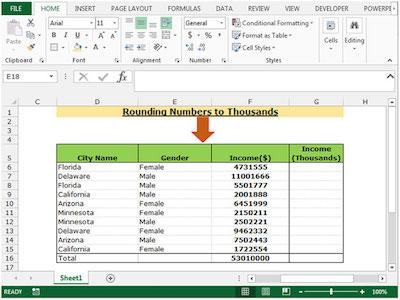The Best Tips And Tricks To Round A Number In Microsoft Excel

The truth is that when you need to round numbers, you can do it alone. While you may struggle a bit, you know you can count on our online rounding calculators to perform these operations for you. This way, you can be sure they are correct.

However, the problem is when you are dealing with big data. As you probably already know, most businesses need to deal with high volumes of data that, sometimes, need to be rounded. So, as you can imagine, it would be impossible to keep doing these roundings one by one by hand or even using our online calculators. For that, you can use software that allow you to perform all the roundings you need fast, quickly, and accurately. One of the most popular software used to round numbers is Microsoft Excel.

So, today, we decided to share some tips and tricks with you so you can easily round a number in Microsoft Excel.

The Best Tips And Tricks To Round A Number In Microsoft Excel

There’s no question that businesses need to deal with decimals. If you think about Pi which is 3.1415926, most of our work tends to be done using only 3.14.

Imagine that you have the decimal 92.45477919 in the cell A2. You probably already know that when you want to decrease the decimal points, you can simply go to the Home tab and then hit the Decrease Decimal until you reach the number of decimals you want. However, there is a much simpler and faster way to do this which is incredibly useful when you’re dealing with big data.

Looking to round to the nearest hundred?

So, the best way to do this is to simply use a formula. Excel provides three formulas for Rounding Numeric numbers:

• ROUND (value, num of decimal digits)
• ROUNDUP(value, num of decimal digits)
• ROUNDDOWN (value, num of decimal digits)

#1: ROUND Function In Excel:

Simply put, the goal of using this function is to limit the decimal value.

Notice that this formula takes 2 arguments: the first one which is the number that you want to round, and the second one which is the number of decimals you want to have.

Easily round your number to the nearest whole.

Getting back at our example above, let’s say that you want to get in cell B2 only 1 decimal digit. In this case, you just need to write:

=ROUND(A2,1)

Notice that if the second decimal digit was greater than or equal to 5 than ROUND function would increase the first decimal by 1. You can see it in Cell B4 in the above image.

#2: ROUNDUP Function In Excel:

Just like the previous function, the ROUNDUP function also limits the decimal points that are shown. However, there is a substantial difference: it always increases the last decimal digit by one.

Discover how to round numbers to the nearest tenth.

This formula also takes two arguments: the first one which is the number that you need to be rounded, and the second one refers to the number of decimals.

So, putting this into practice, let’s say that you want your cell C2 to have only 1 decimal digit but you want to increase it by one to compensate the leftover digits. So you need to write this formula in C2:

=ROUNDUP(A2,1)

#3: ROUNDDOWN Function In Excel:

The ROUNDDOWN function is very similar to the ROUNDUP function that we just showed you. The main difference is that it prevents the rounding up of a number.

Imagine that you write in the cell D2 the following formula:

=ROUNDOWN(A2,1)

As you can see, 92.454779 is now 92.4. Similarly, all numbers in column D are shown as they depend on defined decimal digits.